# Modeling Alpha Half-life

### Integrating the tunneling barrier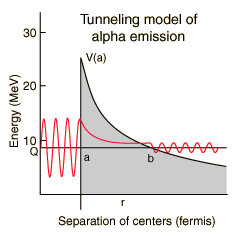A number of parameters must be calculated to model the barrier penetration which leads to alpha emission . The nuclear influence is assumed to stop sharply when the emitted alpha and the reduced nucleus are just touching each other. Using the nuclear radius relationship, this distance for polonium-212 is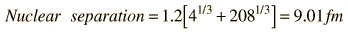For a nucleus with atomic mass A, the above relationship is used with A-4. For A = , then r = a = fermis. The height of the barrier at the above distance is calculated as the Coulomb potential of point charges. The charge of the remaining nucleus has been reduced by two by the alpha emission, so that barrier height V(a) is: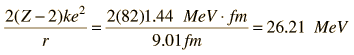and for Z = it is MeV

The distance at which the Coulomb potential drops to the level of energy of the observed polonium-212 alpha isFor an alpha energy of MeV, the distance is r = b = fermi.

So the width of the polonium-212 barrier is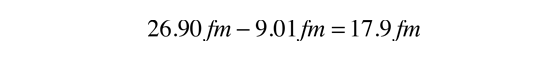and the width for the currently modeled nucleus is fermi.

In addition to the tunneling probability calculated below, the alpha emission rate depends upon how many times an alpha particle with this energy inside the nucleus will hit the walls. The velocity of the alpha can be calculated fromsince an alpha at this energy is nonrelativistic. The frequency of hitting the walls is thenFor the currently modeled nucleus, the frequency is f = x 10^ /s.

To set up the intgration of the tunneling barrier, the first step is to get a differential probability expression. From the tunneling relationship, the probability of tunneling through an infinitesemally thick barrier from r to r+dr is given byThe probability P for penetrating the complete barrier has the form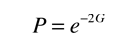where G is called the Gamow factor and has the formThe evaluation of this integral givesWhere x = a/b = Q/V(a). The calculated decay constant is equal to this probability times the frequency of impact on the barrier. In the above expression z=2 for an alpha particle, and Z' = Z-z for the the parent nucleus after emission. For the parameters given, the probability is= x10^

and the resulting decay constant is

λ = fP = x10^

This leads to a calculated halflife of

T1/2 = 0.693/λ = x10^ seconds.

This should be a fairly realistic model of a spherical nucleus. The things it leaves out include the non-spherical nature of many heavy nuclei and the effects of nuclear angular momentum on decay rate. The assumption of a spherical shape is a particular problem for heavy nuclei (A > 230) since they are known to be strongly deformed. This is a serious problem since a 4% change in radius can yield a factor of 5 difference in halflife. Sometimes the measured halflife is used to make implications about the radius instead of vice versa.

 Discussion of polonium alpha decay
Index

Alpha Decay Concepts

References
Rohlf
Sec 7-4

Eisberg & Resnick
Sec 16-2

Krane
Sec 8.4

 HyperPhysics***** Nuclear R Nave
Go Back